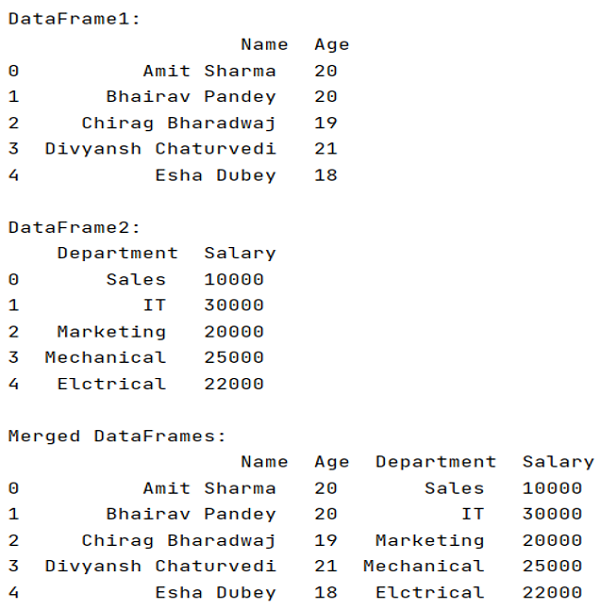# How to merge two DataFrames by index?

Given two DataFrames, we have to merge them by index.
Submitted by Pranit Sharma, on May 17, 2022

DataFrames are 2-dimensional data structures in pandas. DataFrames consist of rows, columns, and the data. DataFrame can be created with the help of python dictionaries or lists but in the real world, CSV files are imported and then converted into DataFrames. Sometimes, DataFrames are first written into CSV files. Here, we are going to merge two DataFrames by index, for this purpose we are going to use pandas.DataFrame.merge() method.

## pandas.DataFrame.merge() Method

When we want to update a DataFrame with the help of another DataFrame, we use pandas.DataFrame.merge() method. This method is used to merge two DataFrames based on an index.

Syntax:

```DataFrame.merge(
right,
how='inner',
on=None,
left_on=None,
right_on=None,
left_index=False,
right_index=False,
sort=False,
suffixes=('_x', '_y'),
copy=True,
indicator=False,
validate=None
)
```

To work with pandas, we need to import pandas package first, below is the syntax:

```import pandas as pd
```

Let us understand with the help of an example.

```# Importing pandas package
import pandas as pd

# Creating a Dictionary
dict1 = {
'Name':['Amit Sharma','Bhairav Pandey','Chirag Bharadwaj','Divyansh Chaturvedi','Esha Dubey'],
'Age':[20,20,19,21,18]
}

dict2 = {
'Department':['Sales','IT','Marketing','Mechanical','Elctrical'],
'Salary':[10000,30000,20000,25000,22000]
}

# Creating a DataFrame
df1 = pd.DataFrame(dict1)
df2 = pd.DataFrame(dict2)

# Display DataFrame
print("DataFrame1:\n",df1,"\n")
print("DataFrame2:\n",df2,"\n")

# Merging two DataFrames
result = pd.merge(df1,df2,left_index=True, right_index=True)

# Display Result
print("Merged DataFrames:\n",result)
```

Here, we have passed two parameters, left_index and right_index which generally means whether to use the index from left and right DataFrame or not, we want the DataFrames to be merged on the basis of index, hence we will set left and right index as True.

Output:What's New (MCQs)

Top Interview Coding Problems/Challenges!

IncludeHelp's Blogs

Languages: » C » C++ » C++ STL » Java » Data Structure » C#.Net » Android » Kotlin » SQL
Web Technologies: » PHP » Python » JavaScript » CSS » Ajax » Node.js » Web programming/HTML
Solved programs: » C » C++ » DS » Java » C#
Aptitude que. & ans.: » C » C++ » Java » DBMS
Interview que. & ans.: » C » Embedded C » Java » SEO » HR
CS Subjects: » CS Basics » O.S. » Networks » DBMS » Embedded Systems » Cloud Computing
» Machine learning » CS Organizations » Linux » DOS
More: » Articles » Puzzles » News/Updates